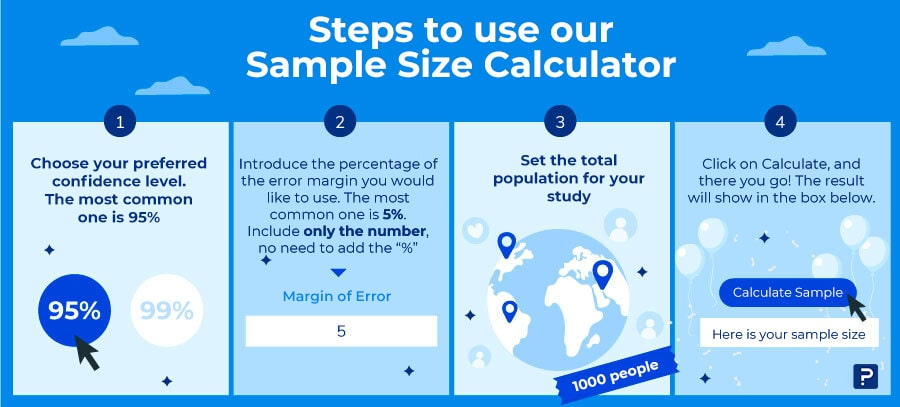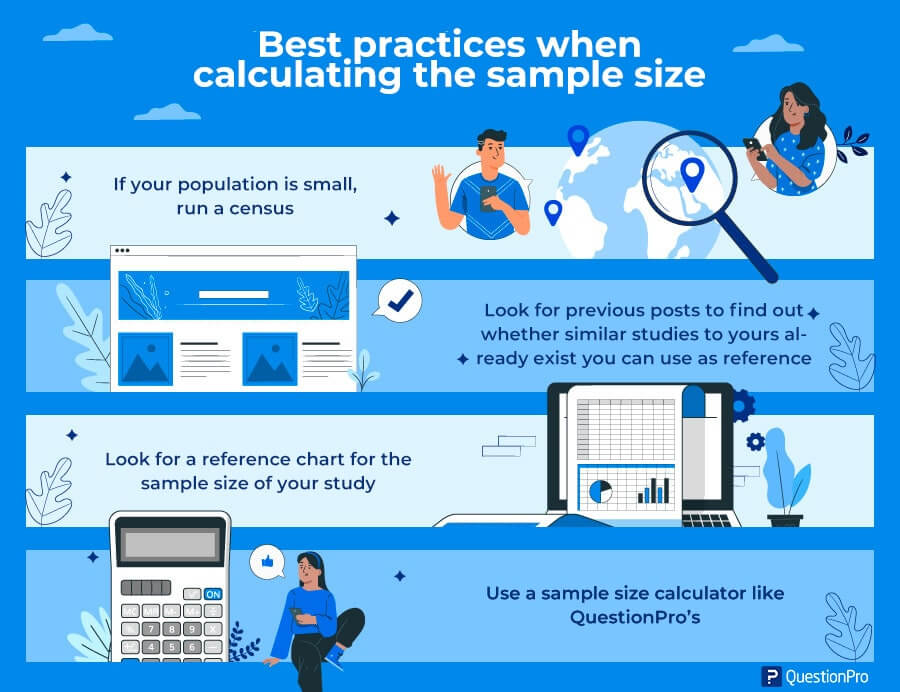Whenever you’re running and planning a research study, there’s nothing as important as knowing the exact number of surveys that you need for the study to be considered valid research material. This is when we need to know what’s the best way to you’ll be able to determine the sample size for your research.

We’re lovers of technology and automation here at QuesionPro. This is why we’ve created a simple sample calculator, so that just a few clicks away, determine the exact number of surveys to run.

If you’d like to know a bit more about how to use this sample calculator, please read the following part, and you’ll become an expert in Sample Calculation.

## Sample size calculator for your research

With this calculator, you’ll be able to quickly and effectively measure the sample size of your next research. Surely, using it will allow you to save a lot of time. Take the most advantage out of it and use it whenever needed.

Sample Size Calculator

Confidence Level: 95% 99%
Margin of Error:
Population:
##### Sample Size:

Confidence Level: Indicates the degree of confidence that the data is representative of the entire population.

Margin of Error: It is the percentage of error that may exist in your sample. The larger the margin of error, the lower the confidence level of the results.

Population: Total number of people your universe represents.

## How to use our sample calculator?

Using our calculator is fairly easy. However, we’d like to share the following tips and notes for you to fully understand the entire process behind this useful, nearly magical tool.### Steps to use our sample size calculator

1. Choose your preferred confidence level. The most common one is 95%.
2. Introduce the percentage of the error margin you would like to use. The most common one is 5%. Include only the number, no need to add the “%”.
3. Set the total population for your study.
4. Click on Calculate, and there you go! The result will show in the box below.

Remember that the sample will be representative because it will have the right margin and confidence level, so follow the following suggestions:

1. Determine the confidence level you would like to obtain. That’s the degree of certainty (or probability) in terms of percentage with which it is pretended to estimate a parameter through a sample statistic. The most effective and common confidence level is 95%.
2. Select the margin of sampling error, which is an indicator of the reliability of the study and the accuracy of your outcomes. The margin of error is put as a percentage that indicates how the outcomes are within an approximate percentage of the values obtained. It’s often 5% or less.
3. Set the total population, you can take help from official data of your community, or an approximate number. For instance, Bogotá, Colombia, has 7743955 inhabitants according to the 2018 census.

If you set the sample calculator to a confidence level of 95%, an error margin of 5%, and a total population of 7743955, the sample size would be 385. Now let’s break these concepts down a bit more:

### The margin of error or confidence interval

The margin of error, also called confidence interval, is the negative or positive number that is generally reported in the outcome of a survey.

For example, if you set the margin of error to 4 and 47% of your sample picks an answer, you can be certain that if you had formulated the question to the entire population, from 43% (47% - 4) to 51% (47% + 4) would have chosen that answer.

To facilitate this process, you can too use our margin of error calculator.

### Confidence level

The confidence level is how frequently the real percentage of the population would choose a specific answer. A confidence level of 95% means you can be 95% certain; a confidence level of 99% means you can be 99% certain. Most researchers use a 95% confidence level.

For instance, if you ask a sample of 1000 people in a city what are their preferences in terms of soda, and 60% claim it’s Brand A, you can be certain that 40% - 80% of people in the city prefer that brand, but you can’t be that certain that 59% - 61% of the people in the city prefer that brand.

Three factors help determine the confidence of your research:

• Sample size
• Percentage or variability
• Total population

### Sample size

The greater the sample size, the more certain one can be that the responses represent the population. This means that for a determined confidence level, the bigger the sample, the smaller the margin of error (or confidence interval).

Nonetheless, this relationship is not linear. In other words, doubling the sample size, will not reduce at half the margin of error.

### Percentage or variability

The accuracy also depends on the percentage of the sample that picks a response in particular. If 99% of your sample said “Yes” and 1% “No”, the probability of an error is remote, regardless of the sample size. However, if percentages are 51% and 49% respectively, the likelihood of an error is broader.

Determining the sample size for a determined accuracy level, you must use the worst possible percentage (50%). Also, you must use this percentage when determining a general level of accuracy for a given sample.

To determine the margin of error for a specific response in your sample, you can use the percentage by picking that answer and obtaining a narrower interval.

In research, it’s common to go for the maximum variability option, especially when there are no backgrounds for a subject. For these cases a standard value of p=q=0.5 or 50%.

### Total population

It’s unlikely to know the exact size of a specific population. However, it is not an issue.

The mathematical probabilities show us that the total population is irrelevant unless the sample size exceeds a small percentage of the total population you’re researching.

This means that a sample of 500 people is just as useful to research a state of 15,000,000 or a city of 100,000.

The calculation of the confidence interval infers that you have a random and confident population sample.

If your sample is not truly random, you cannot trust those intervals. Samples that are not random are usually a result of an error or limitation in the sampling procedure.

An example of this error would be calling people to their homes during the day, and put aside anyone who’s not at home (like most workers in the world). You cannot assume that non-workers (or home-workers) represent accurately an entire population.

To learn more on sample size calculation, check our guide on: How to calculate sample size?

## Sample calculator formula

Although it may seem like magic, behind our sample size calculator there is a methodology that validates the sample calculation. For this one, we rely on the following formula:

### Sample size = Z2 * (p) * (1-p) / c2

Where:

• Z = confidence level ( 95% or 99%)
• p = .5
• c = Margin of error ( .04 = ±4%)

## Tips for a proper calculation of the sample size

Here you can find a few suggestions to determine the sample size in your next research:### Best practices when calculating the sample size

• If your population is small, run a census.
• Look for previous posts to find out whether similar studies to yours already exist.
• Look for a reference chart for the sample size of your study.
• Use a sample size calculator like QuestionPro’s.

## Importance of calculating the sample size

Samples can offer data that allow inferring over the entire population. It’s through compiled data that researchers may get findings of a population.

Other reasons why it’s important to calculate the sample size, are the following:

• The two main factors that affect the utility and efficacy of a study are the sample size, and the effect size.
• A study must only be run once. There is a real possibility that its outcomes will provide useful information.
• A study that has a sample size too small may produce inconclusive outcomes and may also be considered unethical by exposing subjects to unnecessary risks.
• A study too big will be a waste of resources and may expose more subjects than needed to any related risks.

In summary, the sample size calculation is a very important process within any research project. It must be run during the planning phase of the study, based on the question types and the research design.

It’s also advisable to count with a sample size calculator such as QuestionPro’s for help, to avoid errors when trying to determine the percentage of the population to be researched.

We hope this sample size calculator comes in handy to collect the necessary data that assures success for your research project.

### What to do with your sample?

There! Now you know the exact number of surveys to run for your study to be within a valid range, thanks to the sample size calculated. Do you need an automatic tool for the collection and analysis of your data? At QuestionPro, we can help. What are you waiting for?